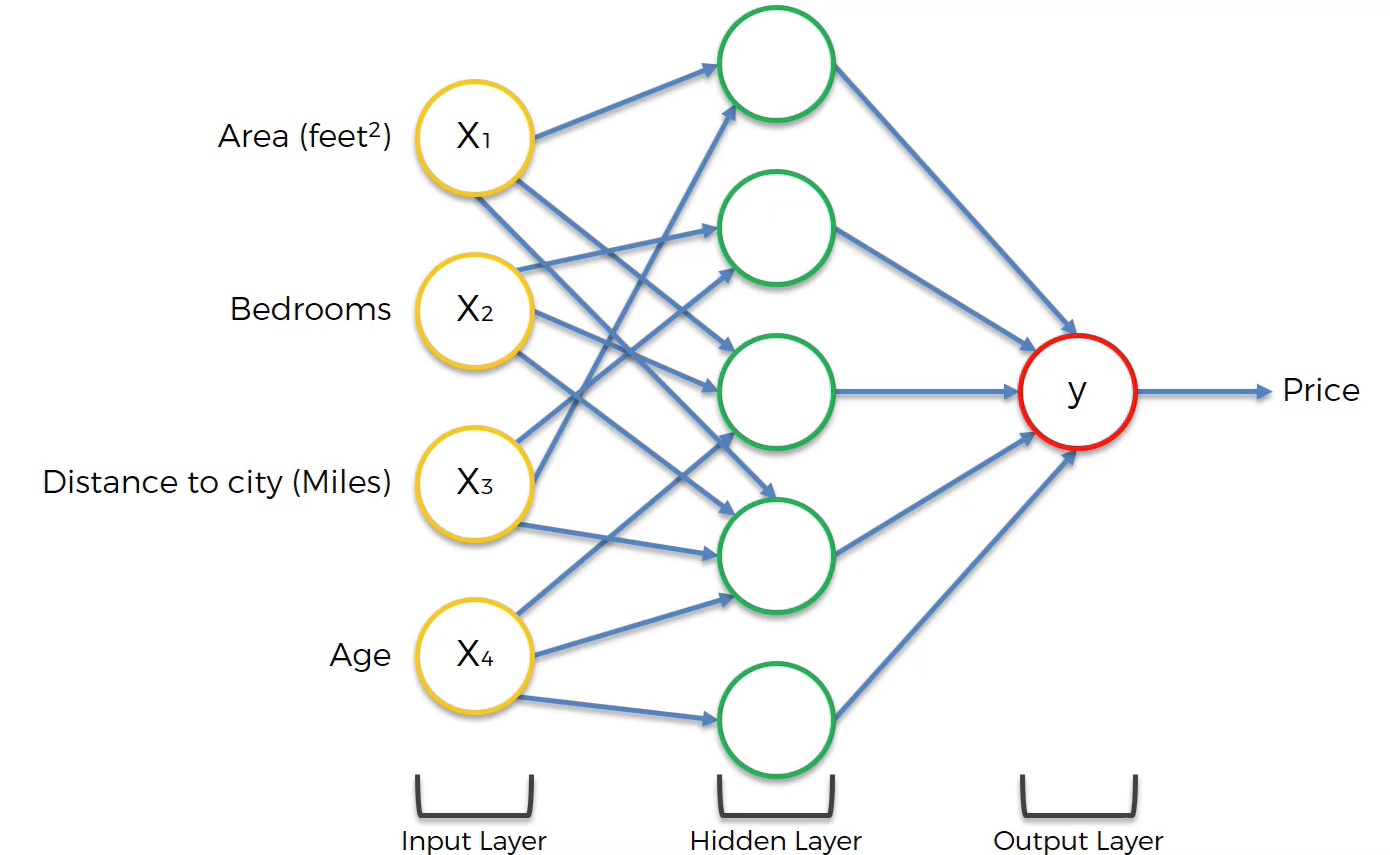# How Do Neural Networks Really Work?

Hey - Nick here! This page is a free excerpt from my \$199 course Python for Finance, which is 50% off for the next 50 students.

In separate articles, we have discussed two of the building blocks for building neural networks:

However, you're probably still a bit confused as to how neural networks really work.

This tutorial will put together the pieces we've already discussed so that you can understand how neural networks work in practice.

## The Example We'll Be Using In This Tutorial

This tutorial will work through a real-world example step-by-step so that you can understand how neural networks make predictions.

More specifically, we will be dealing with property valuations.

You probably already know that there are a ton of factors that influence house prices, including the economy, interest rates, its number of bedrooms/bathrooms, and its location.

The high dimensionality of this data set makes it an interesting candidate for building and training a neural network on.

One caveat about this tutorial is the neural network we will be using to make predictions has already been trained. We'll explore the process for training a new neural network in the next section of this course.

## The Parameters In Our Data Set

Let's start by discussing the parameters in our data set. More specifically, let's imagine that the data set contains the following parameters:

• Square footage
• Bedrooms
• Distance to city center
• House age

These four parameters will form the input layer of the artificial neural network. Note that in reality, there are likely many more parameters that you could use to train a neural network to predict housing prices. We have constrained this number to four to keep the example reasonably simple.

## The Most Basic Form of a Neural Network

In its most basic form, a neural network only has two layers - the input layer and the output layer. The output layer is the component of the neural net that actually makes predictions.

For example, if you wanted to make predictions using a simple weighted sum (also called linear regression) model, your neural network would take the following form:While this diagram is a bit abstract, the point is that most neural networks can be visuailzed in this manner:

• An input layer
• Possibly some hidden layers
• An output layer

It is the hidden layer of neurons that causes neural networks to be so powerful for calculating predictions.

For each neuron in a hidden layer, it performs calculations using some (or all) of the neurons in the last layer of the neural network. These values are then used in the next layer of the neural network.

## The Purpose of Neurons in the Hidden Layer of a Neural Network

You are probably wondering - what exactly does each neuron in the hidden layer mean? Said differently, how should machine learning practitioners interpret these values?

Generally speaking, neurons in the midden layers of a neural net are activated (meaning their activation function returns `1`) for an input value that satisfies certain sub-properties.

For our housing price prediction model, one example might be 5-bedroom houses with small distances to the city center.

In most other cases, describing the characteristics that would cause a neuron in a hidden layer to activate is not so easy.

## How Neurons Determine Their Input Values

Earlier in this tutorial, I wrote "For each neuron in a hidden layer, it performs calculations using some (or all) of the neurons in the last layer of the neural network."

This illustrates an important point - that each neuron in a neural net does not need to use every neuron in the preceding layer.

The process through which neurons determine which input values to use from the preceding layer of the neural net is called training the model. We will learn more about training neural nets in the next section of this course.

## Visualizing A Neural Net's Prediction Process

When visualizing a neutral network, we generally draw lines from the previous layer to the current layer whenever the preceding neuron has a weight above `0` in the weighted sum formula for the current neuron.

The following image will help visualize this:As you can see, not every neuron-neuron pair has synapse. `x4` only feeds three out of the five neurons in the hidden layer, as an example. This illustrates an important point when building neural networks - that not every neuron in a preceding layer must be used in the next layer of a neural network.

## Final Thoughts

In this tutorial, you learned more about how neural networks perform computations to make useful predictions.

Here is a brief summary of what we discussed:

• That predicting housing prices is a useful example to illustrate how neural networks work
• How high-dimensionality data is well-suited to be used with artificial neural nets
• What the most basic form of a neural network looks like
• How the hidden layer of a neural net is its main source of predictive power
• That neurons in a neural net don't necessarily need to use the values from every neuron in the preceding layer